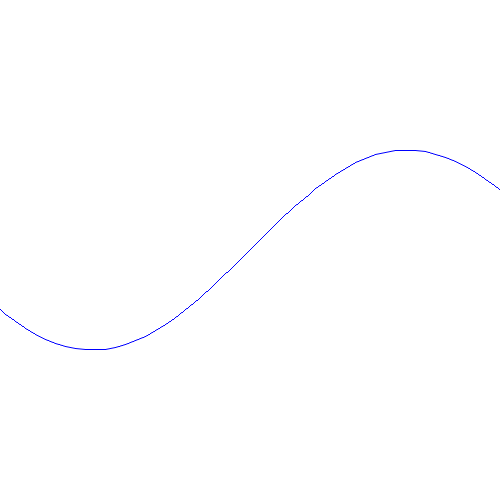## Draw sin curve using Imager

Write sin curve using Imager.

Chart::GGPlot author say to me "It is hard to use graphic basic library for me".

so I want to illustrate basic drawing.

Cairo seems to be good as features but portability is not good.

so I try to plot sin curve by Imager.

Curve is continue of very short lines.

I wrote a graph of sin.

Performance is very fast. Generation of graph is in a second.```use strict;
use warnings;

use Imager;
use Imager::Color;
use Math::Spline;
use Math::Trig 'pi';

my \$xsize = 500;
my \$ysize = 500;
my \$x_offset = 250;
my \$y_offset = 250;

my \$img = Imager->new(xsize => \$xsize, ysize => \$ysize, channels => 4);

my \$scale = 100;

my \$min = -pi();
my \$max = 2*pi();

my \$kannkaku = 0.1;

my \$points = [];
for (my \$x = \$min; \$x < \$max; \$x += 0.1) {
my \$y = sin(\$x);
my \$point = [\$x * \$scale + \$x_offset, \$y * \$scale + \$y_offset];
push @\$points, \$point;
}

# my @ys = map { xx(\$_) } @xs;

# my \$spline = Math::Spline->new(\@xs, \@ys);

# my \$x = 5;
# my \$y = \$spline->evaluate(\$x);

my \$blue = Imager::Color->new( 0, 0, 255 );
# my \$fill = Imager::Fill->new(hatch=>'stipple');

\$img->box(color => Imager::Color->new(255, 255, 255), xmin=>0, ymin=>0, xmax=>400, ymax=>300,
filled=>1);

\$img->polyline(points => \$points, color => \$blue, aa => 1);

\$img->flip(dir => 'v');

# Jpeg、画質 90% で保存
\$img->write( file => 'graph.png', jpegquality => 90 )
or die \$img->errstr;
```I'm Perl Programmer. I LOVE Perl. I want to contribute Perl community and Perl users.